# Place Value of Tenths, Hundreds and Thousandths

Place Value of Tenths, Hundreds and Thousandths• The decimal point is used to separate the whole numbers from numbers that are smaller than one whole.
• Numbers to the right of the decimal point are shown in the decimal place value columns.
• From left to right, we have tenths, hundredths, thousandths, ten thousandths and so on.
• As we move from left to right, each place value column is ten times smaller than the last.

The decimal place value columns from left to right are called tenths, hundredths and thousandths.• 49 / 100   is the decimal number 49 hundredths.
• The hundredths place value column is two places to the right of the decimal point.
• We put the last digit of 49 in the hundredths column and the other digits go in front of this.
• The 9 goes in the hundredths column and the 4 goes in front in the tenths column.
• We should always write a digit in the ones (units) column and because we have no more digits remaining, we write a 0 in front of the decimal point.
• 49 / 100   is written as 0.49.
• The 0 in the ones column tell us that 49 hundredths is smaller than 1 whole.Supporting Lessons

#### How Big are Tenths, Hundredths and Thousandths?# Place Value of Tenths, Hundreds and Thousandths

## What is Decimal Place Value?

Decimal place value columns show the parts of a number that are smaller than one whole. Moving from left to right, each column gets ten times smaller. From left to right, after the decimal point, the columns are called tenths, hundredths, thousandths and so on.

• The first digit after the decimal point is the tenths column.
• The second digit after the decimal point is the hundredths column.
• The third digit after the decimal point is the thousandths column.
• The fourth digit after the decimal point is the ten thousandths column.

Here is a blank decimal place value chart which shows the place value columns and the size of the fractions they represent.After the decimal point, the place value columns are called tenths, hundredths, thousandths, ten thousandths and so on.

The digit in the tenths column represents a fraction out of ten,   1 / 10  .

The digit in the hundredths column represents a fraction out of one hundred,   1 / 100  .

The digit in the thousandths column represents a fraction out of one thousand,   1 / 1000  .

As we move from one column to the column on its right, the digit represented in ten times smaller.

Here is an example of finding the place value of a decimal number. A Decimal place value chart is used to show how different decimal digits can be written as a fraction.

We have the decimal number 4.5326.

Ones Decimal Point Tenths Hundredths Thousandths Ten Thousandths
4 . 5 3 2 6
4 . 5 / 10 3 / 100 2 / 1000 6 / 10000

4 is the digit before the decimal point and so, it is a whole number.

The 5 is in the tenths column and is worth   5 / 10  .

The 3 is in the hundredths column and is worth   3 / 100  .

The 2 is in the thousandths column and is worth   2 / 1000  .

The 6 is in the ten thousandths column and is worth   6 / 10000  .

The further to the right the decimal digit, the smaller its value. The decimal digits closest to the decimal point are greatest in value.

Tenths are larger than hundredths, which are larger than thousandths and so on.

## What are Tenths?

Tenths are written in the first digit to the right of the decimal point. They are ten times smaller than ones. Tenths are written as a fraction out of 10.

A tenth is the same as 1 ÷ 10. A tenth is ten times smaller than a whole.

Here is one whole.And here is the size of one tenth. One tenth fits into one whole ten times.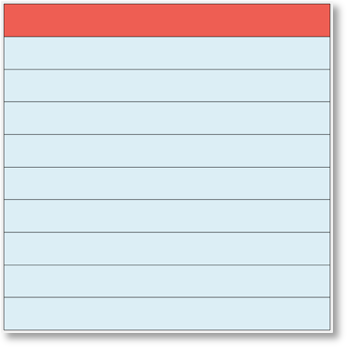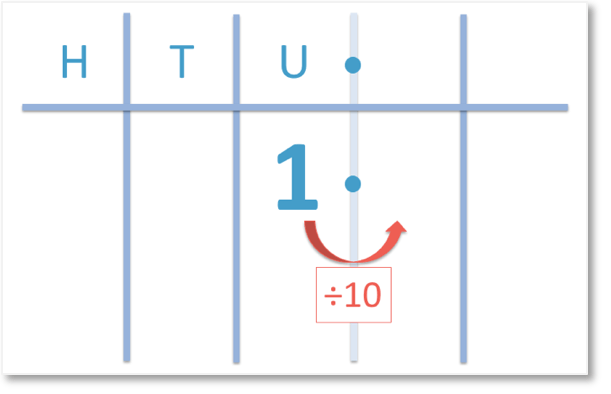1 ÷ 10 = 0.1 and so, 1 tenth is equal to 0.1.The tenths place value column is the first digit shown after the decimal point.

Here we can see the effect of dividing by 10 on a place value chart to get to one tenth.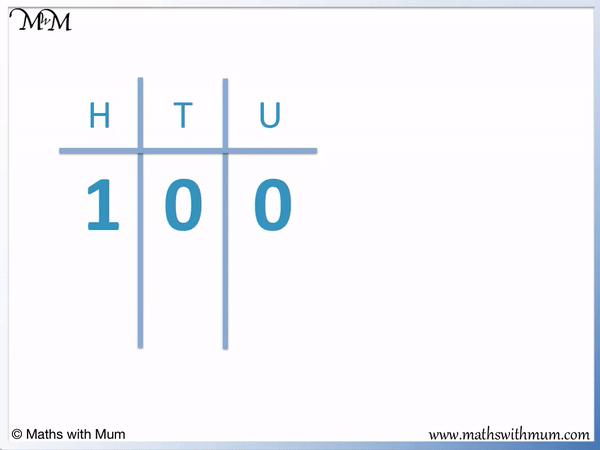Digits in the tenths place value column can always be written as a fraction out of 10.

To write a tenth as a decimal, write the numerator of the fraction in the tenths column, after the decimal point.

Here is an example of 3 tenths. 3 tenths is written as   3 / 10  .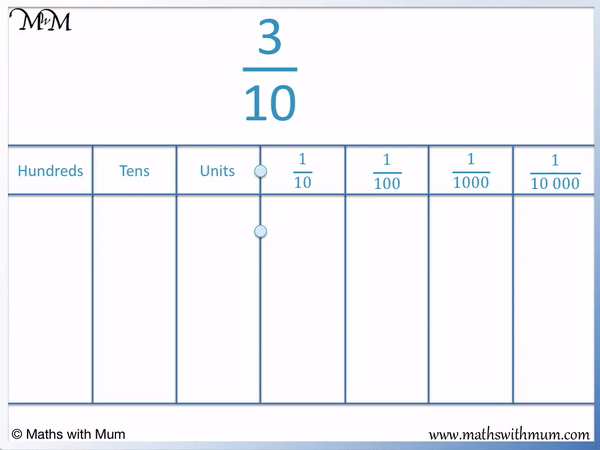3 is the numerator of the fraction. The fraction is out of 10 so we write the 3 in the tenths column, after the decimal point.

Since we do not have any ones, we put a zero before the decimal point.

The fraction   3 / 10   can be written as a decimal as 0.3.

0.3 is the same as   3 / 10  , which means 3 divided by 10.

It is important not to confuse the tens place value column with the tenths place value column. The tenths place value column is one place to the right of the decimal point, whereas the tens column is two places to the left of the decimal point. Tens are ten times larger than one whole, whereas tenths are ten times smaller than one whole.

## What are Hundredths?

Hundredths are the second digit to the right of the decimal point. They are written as a fraction out of 100. Hundredths are one hundred times smaller than numbers in the ones column.

1 ÷ 100 = 0.01. One hundredth is written as a decimal as 0.01.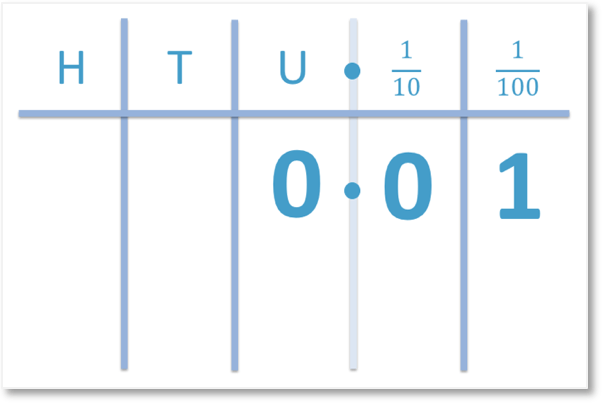One hundredth is one hundred times smaller than a whole. One hundredth is ten times smaller than one tenth.

Here is a tenth.Here is one hundredth. One hundred hundredths make a whole.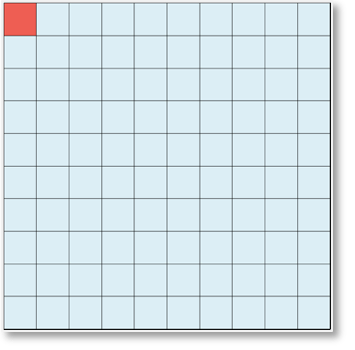One hundredth is one tenth divided by ten again. Ten hundredths make a tenth and one hundred hundredths make one whole.Here is an example of writing hundredths as a decimal. Here is   8 / 100  , which is said as eight hundredths.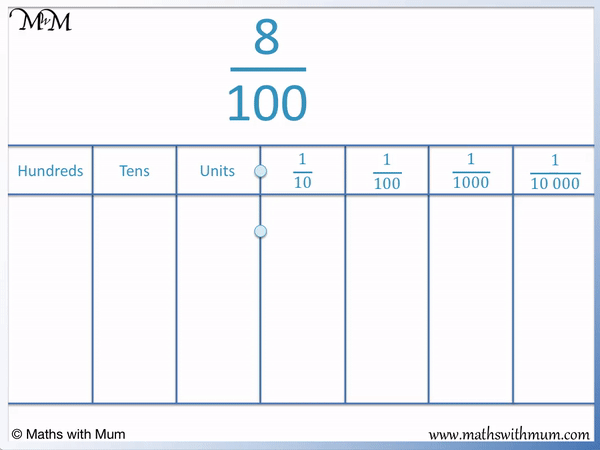The 8 is a fraction out of 100. Therefore this fraction is worth 8 hundredths. We write the 8 in the hundredths column, two places to the right of the decimal point.

We need to put zeros in the tenths column and ones column to show there is nothing there.

8 / 100   is written as a decimal as 0.08.

We need to put a zero in the ones column to show that there is no whole number component and we need to put a zero between the decimal point and the 8 to show that there is no tenths.

If we didn’t put a zero between the decimal point and the 8, we would have written 0.8, which is 8 tenths not 8 hundredths.

Here is another example of writing a fraction in the hundredths as a decimal.

We have   49 / 100  .To write a hundredth as a decimal, take the last digit of the numerator and put it 2 places to the right of the decimal point. Then copy the rest of the digits from the numerator in the same order in front of this.

The last digit of the numerator is a 9. We put the 9 in the hundredths column, 2 places to the right of the decimal point and then put the 4 in front of this in the tenths column.

There are no other digits, so we write a 0 in the ones column.

The fraction   49 / 100   can be written as a decimal as 0.49.

There are one hundred hundredths in one whole. There are 49 hundredths in 0.49.

It is important to know the difference between hundreds and hundredths. Hundreds are one hundred times larger than one whole, whereas hundredths are one hundred times smaller than one whole. The hundreds place value column is three places to the left of the decimal point and the hundredths place value column is two places to the right of the decimal point.

## What are Thousandths?

Thousandths are the third digit to the right of the decimal point. They are written as a fraction out of 1000. Thousandths are one thousand times smaller than numbers in the ones column.

Here is an example of 206 thousandths. Written as a fraction, this number is   206 / 1000  .To write a number in the thousandths as a decimal, write the last digit of the numerator 3 places to the right of the decimal point. Write the other digits in the numerator in the same order in front of it.

The last digit of 206 is a 6. We place the 6 in the thousandths column, three places to the right of the decimal point. We then place the 2 and the 0 in the same order in front of this.

The fraction   206 / 1000   is written as a decimal as 0.206.

Here is another example of writing a fraction in the thousandths as a decimal.

We have   52 / 1000  .The digit of 2 is the last digit in the numerator of the fraction. Since the fraction is out of 1000, we write the 2 in the thousandths column, three places to the right of the decimal point.

We put the 5 in front of this in the hundredths column.

The are no other digits so we must put a 0 in the tenths column and a 0 in the ones column.

It is important to write 0.052 instead of 0.52 as these decimal numbers are not the same size.

The difference between thousands and thousandths is that thousands are one thousand times larger than one whole, whereas thousandths are one thousand times smaller than one whole. The thousands place value column is 4 places to the left of the decimal point and the thousandths place value column is 3 places to the right of the decimal point.

## Decimal Place Value

One thousandth is ten times smaller than one hundredth, which is ten times smaller than one tenth, which is ten times smaller than one whole.

One tenth is 10 times smaller than one whole.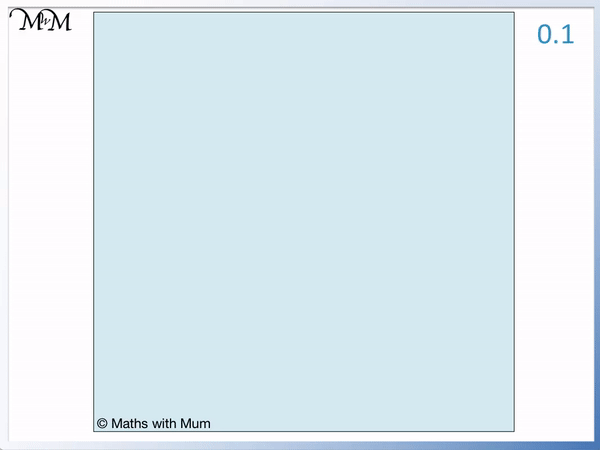One hundredth is 100 times smaller than one whole.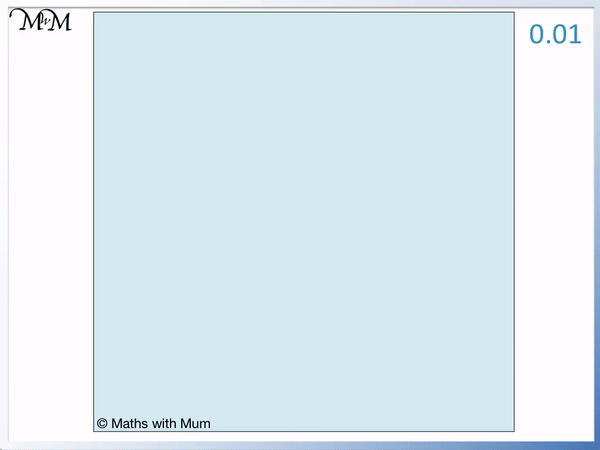One thousandth is 1000 times smaller than one whole.

Here is an example of visualising the size of a decimal number.

0.28 is shown below in its place value columns.0.28 can be written as 28 hundredths, which means 28 divided by 100.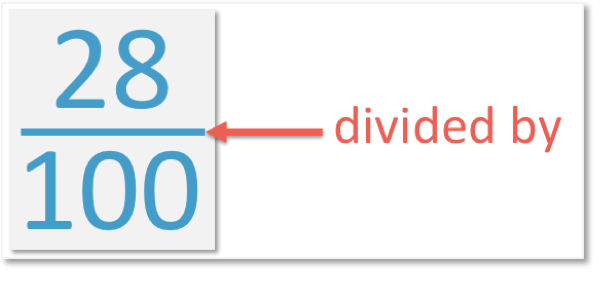Here are 28 hundredths shown in the image below. Each square is the size of one hundredth.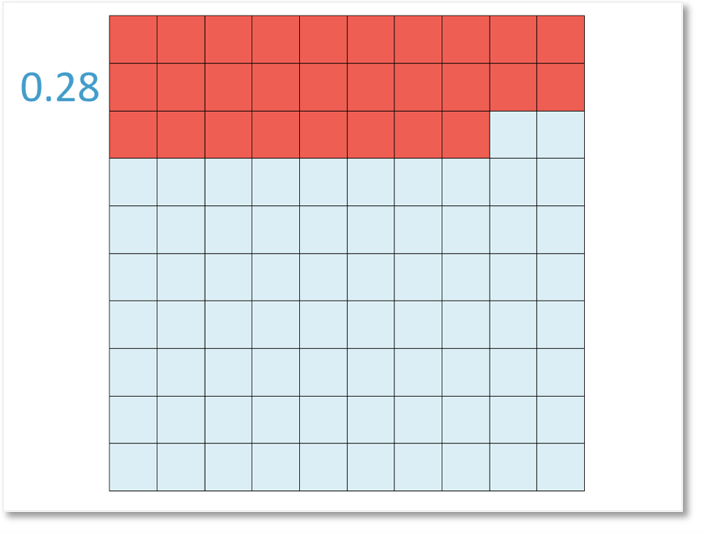0.28 contains 2 tenths and 8 hundredths. We can also show it as 2 tenths and 8 hundredths.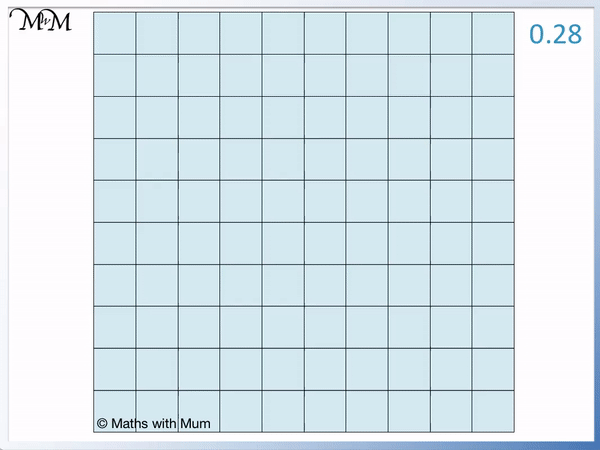28 hundredths is the same size as 2 tenths and 8 hundredths combined.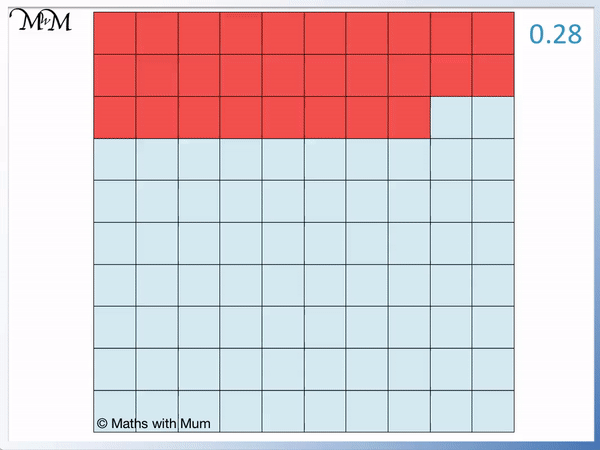Here is another example of visualising the size of the decimal number 0.64.

We have 64 hundredths in total, so 64 squares are shown.0.64 is worth 64 hundredths, which is the same as 6 tenths and 4 hundredths combined.We can see that over half of the whole square is shaded in.

0.5 is one half and 0.64 is a little larger than this.

When teaching decimals, children can often find it difficult to understand the relative size of tenths and hundredths. It is useful to show diagrams or even to use base ten blocks to help understand the comparative sizes.

Here is an example of dividing 200 by 10 several times until we get to 2 tenths.200 ÷ 10 = 20 and 20 ÷ 10 = 2.

We can keep dividing to get decimal numbers.

2 ÷ 10 = 0.2, which we call 2 tenths. 2 tenths is simply 2 divided by 10.

We can show the size of 2 tenths by taking one whole, dividing it by ten and shading two of these tenths.We can divide 2 tenths by ten one more time to make 2 hundredths.

0.2 ÷ 10 = 0.02, which can be seen on the place value chart below.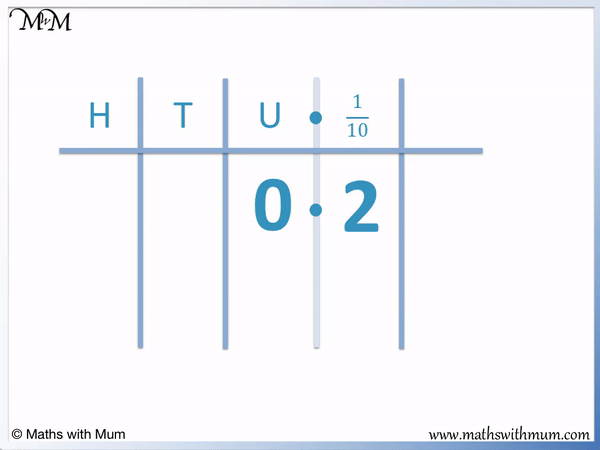2 hundredths is the same as taking one whole, dividing it into 100 pieces and shading in 2 of these.Now try our lesson How to Write Decimals as Fractions where we learn how to write a decimal number as a fraction.